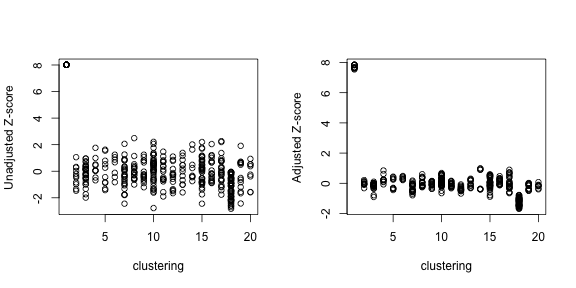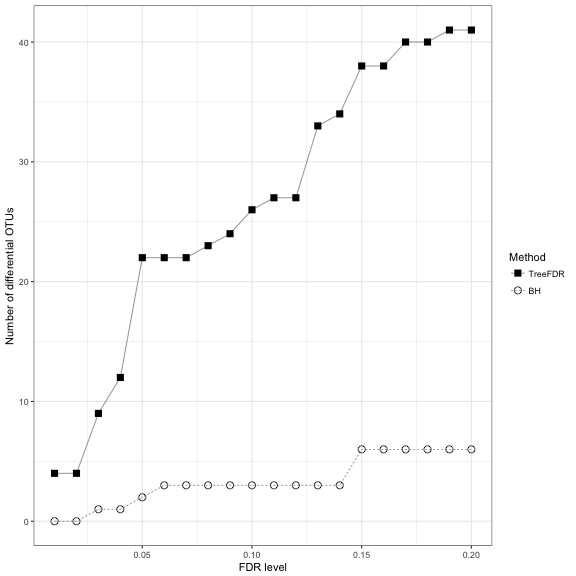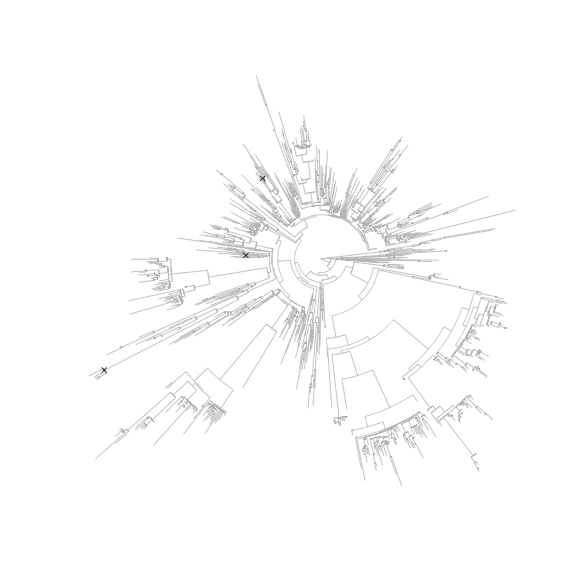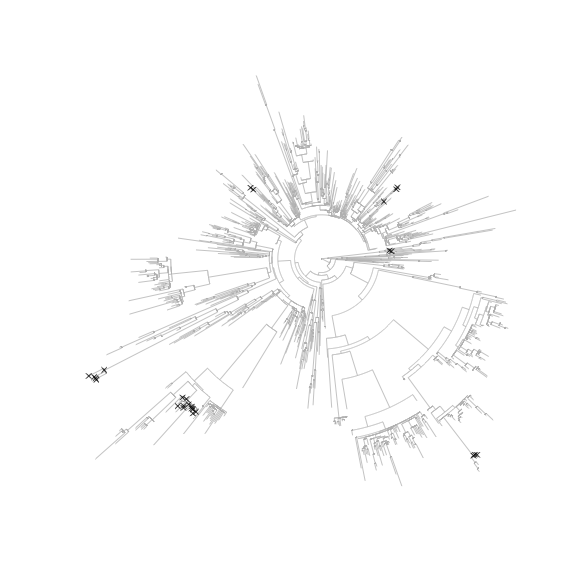In this vignette, we will illustrate the use of TreeFDR through simulated data sets and a real data set (alcohol intake and microbiome association). We first begin with a simulated data set with 50 case and 50 control samples. We simulate a random coalescent tree of 400 OTUs. The OTU counts are generated based on a Dirichlet-multinomial model, where the composition parameters are estimated from a real throat microbiome data set. We allow an average sequencing depth of 10,000 reads per sample. Next, we simulate differential OTUs by multiplying the case counts by a scalar. We explore different scenarios by calling the simulation function 'SimulateData' included in the package. In the following scenario ('S1'), the tree is partitioned into 20 clusters, and 2 clusters of OTUs are differentially abundant with the same fold changes in the same cluster. In this scenario, the phylogenetic tree is highly informative.

`````` require(StructFDR)
require(ape)
require(ggplot2)
require(reshape)
``````
``````# Generate artificial data
set.seed(12345)
data(throat.parameter)
data.obj <- SimulateData(nCases = 50, nControls = 50, nOTU = 400, nCluster = 20,
depth = 10000, p.est = throat.parameter\$p.est, theta = throat.parameter\$theta,
scene = 'S1', signal.strength = 4)
Y <- data.obj\$y
X <- data.obj\$X
tree <-data.obj\$tree
clustering <- data.obj\$clustering
beta.true <- data.obj\$beta.true
meta.dat <- data.frame(grp=factor(data.obj\$y))
``````

Our procedure requires the user to specify a test function, which outputs the p values and the effect directions, and a permutation function, which permutes the data to approximate the null distribution. Here we use a very simple test and permutation function (Wilcoxon rank-sum test, group label permutation). For a more complex example (residual permutation, adjusting covariates), we refer the readers to the function 'MicrobiomeSeqTreeFDR' included in the package.

``````# Define test function based on a simple Wilcoxon rank-sum test
test.func <- function (X, Y) {
Y <- as.numeric(factor(Y))
obj <- apply(X, 1, function(x) {
p.value <- suppressWarnings(wilcox.test(x ~ Y)\$p.value)
e.sign <- sign(mean(x[Y == 2]) - mean(x[Y == 1]))
c(p.value, e.sign)
})
return(list(p.value=obj[1, ], e.sign=obj[2, ]))
}

# Define permutation function, simple group label permutation
perm.func <- function (X, Y) {
return(list(X=X, Y=sample(Y)))
}

# Call TreeFDR
tree.fdr.obj <- TreeFDR(X, Y, tree, test.func, perm.func)
``````
``````## Test on original data sets  ...
## Test on permuted data sets  ...
## Perform ordinary BH-based FDR control ...
## Estimating hyperparameter ...
## Structure-based adjustment ...
## Done!
``````
``````# Performance measure: compare TreeFDR and BH

# Number of truly differential OTUs
sum(beta.true != 0)
``````
``````##  78
``````
``````# Empirical power for treeFDR and BH procedure respectively
(tree.emp.pwr <- sum(tree.p.adj <= 0.05 & beta.true != 0) / sum(beta.true != 0))
``````
``````##  0.3333333
``````
``````(BH.emp.pwr <- sum(BH.p.adj <= 0.05 & beta.true != 0) / sum(beta.true != 0))
``````
``````##  0.1923077
``````
``````# Empirical FDR for treeFDR and BH procedure respectively
(tree.emp.fdr <- sum(tree.p.adj <= 0.05 & beta.true == 0) / sum(tree.p.adj <= 0.05))
``````
``````##  0
``````
``````(BH.emp.fdr <- sum(BH.p.adj <= 0.05 & beta.true == 0) / sum(BH.p.adj <= 0.05))
``````
``````##  0
``````
``````# The effects of moderation by plotting unadjusted Z-score against adjusted Z-score.
par(mfrow=c(1, 2))
``````In this simulation, we can see 'TreeFDR' can increase the power of detecting differential OTUs by borrowing information from neighboring OTUs. The Z-scores of OTUs from the same clusters are smoothed with respect to the underlying phylogeny. The strength of the smoothness depends on the 'informativeness' of the phylogeny. In the above simulation setting, the phylogeny is highly informative. Thus we see strong smoothing/moderation effects.

Other simulation settings can be explored by calling the simulation function 'SimulateData'. For example, 'S2' corresponds to the scenarios of two clusters with variable coefficients, so the phylogenetic information becomes weaker compared to 'S1'. In 'S3', we allow 10 small differentially abundant clusters out of 100 clusters instead of 2 relatively large clusters as in 'S1' and 'S2'. 'S4' and 'S5' represent the non-informative phylogeny scenarios. In 'S4', we let the OTUs within the same cluster have opposite effects. Thus the phylogeny is completely non-informative or even has adverse effects. Finally, 'S5' simulates 10% differentially abundant OTUs randomly drawn without respect to the underlying phylogeny.

To study the effect of within-clade non-differential OTU, we can simulate a certain percentage of non-differential OTUs on the basis of 'S1'. This could be achieved by using the parameter 'zero.pct' (percentage of non-differential OTUs):

``````# Generate artificial data
set.seed(12345)
data.obj <- SimulateData(nCases = 50, nControls = 50, nOTU = 400, nCluster = 20,
depth = 10000, p.est = throat.parameter\$p.est, theta = throat.parameter\$theta,
scene = 'S1', signal.strength = log(4), zero.pct = 0.1, balance = TRUE)
Y <- data.obj\$y
X <- data.obj\$X
tree <-data.obj\$tree
clustering <- data.obj\$clustering
beta.true <- data.obj\$beta.true
``````

We next demonstrate our procedure using a real data set from the study of the effects of dietary intake on the gut microbiome composition. We use the alcohol intake data to illustrate our method. Use '?alcohol' to see the details of the data set.

``````data(alcohol)
set.seed(12345)

X <- alcohol\$X
Y <- alcohol\$Y
tree <- alcohol\$tree
tree.fdr.obj <- TreeFDR(X = X, Y = Y, tree = tree, test.func = test.func, perm.func = perm.func, B = 100)
``````
``````## Test on original data sets  ...
## Test on permuted data sets  ...
## Perform ordinary BH-based FDR control ...
## Estimating hyperparameter ...
## Structure-based adjustment ...
## Done!
``````
``````# Compare treeFDR and BH procedure

# Empirical power
``````
``````##  26
``````
``````sum(BH.p.adj <= 0.1)
``````
``````##  3
``````

We next inspect the taxonomical lineages of the identified OTUs. Many OTUs identified by TreeFDR have the same or similar lineages.

`````` # TreeFDR
alcohol\$otu.name[rownames(alcohol\$X)[tree.p.adj <= 0.1], c('Phylum', 'Class', 'Family')]
``````
`````` ##       Phylum           Class                Family
## 8223  "Firmicutes"     "Clostridia"         "Lachnospiraceae"
## 8671  "Firmicutes"     "Clostridia"         "Lachnospiraceae"
## 17142 "Firmicutes"     "Clostridia"         "Lachnospiraceae"
## 2920  "Proteobacteria" "Other"              "Other"
## 7882  "Proteobacteria" "Betaproteobacteria" "Other"
## 5103  "Proteobacteria" "Other"              "Other"
## 7890  "Proteobacteria" "Betaproteobacteria" "Other"
## 1284  "Proteobacteria" "Other"              "Other"
## 851   "Bacteroidetes"  "Bacteroidetes"      "Other"
## 12915 "Other"          "Other"              "Other"
## 2155  "Bacteroidetes"  "Other"              "Other"
## 13268 "Bacteroidetes"  "Bacteroidetes"      "Other"
## 2535  "Bacteroidetes"  "Bacteroidetes"      "Other"
## 152   "Bacteroidetes"  "Bacteroidetes"      "Other"
## 4816  "Other"          "Other"              "Other"
## 1650  "Bacteroidetes"  "Other"              "Other"
## 16869 "Bacteroidetes"  "Other"              "Other"
## 4871  "Bacteroidetes"  "Other"              "Other"
## 14309 "Bacteroidetes"  "Bacteroidetes"      "Other"
## 9939  "Bacteroidetes"  "Bacteroidetes"      "Bacteroidaceae"
## 15028 "Bacteroidetes"  "Bacteroidetes"      "Bacteroidaceae"
## 7038  "Bacteroidetes"  "Bacteroidetes"      "Bacteroidaceae"
## 403   "Firmicutes"     "Other"              "Other"
## 11686 "Firmicutes"     "Erysipelotrichi"    "Erysipelotrichaceae"
## 13445 "Firmicutes"     "Clostridia"         "Lachnospiraceae"
## 14840 "Firmicutes"     "Clostridia"         "Lachnospiraceae"
``````
`````` # BH
alcohol\$otu.name[rownames(alcohol\$X)[BH.p.adj <= 0.1], c('Phylum', 'Class', 'Family')]
``````
`````` ##       Phylum           Class                Family
## 10825 "Firmicutes"     "Clostridia"         "Ruminococcaceae"
## 7882  "Proteobacteria" "Betaproteobacteria" "Other"
## 2906  "Firmicutes"     "Clostridia"         "Other"
``````

We compare the numbers of differential OTUs at different FDR cutoffs for TreeFDR and BH. We can see that TreeFDR consistently recovers much more OTUs than BH procedure at all FDR levels compared.

``````dat <- sapply(seq(0.01, 0.2, len=20), function (x) {
})
colnames(dat) <- seq(0.01, 0.2, len=20)
dat <- melt(dat)
colnames(dat) <- c('Method', 'FDR_cutoff', 'OTU_number')
dat\$Method <- factor(dat\$Method, levels=c('TreeFDR', 'BH'))
ggplot(dat, aes_string(x='FDR_cutoff', y='OTU_number', group = 'Method',
shape='Method', linetype='Method')) +
geom_line(size=0.2) +
geom_point(size=3) +
ylab('Number of differential OTUs') +
xlab('FDR level') +
scale_shape_manual(values=c(15, 1)) +
theme_bw()
``````Finally, we plot the identified OTUs on the phylogenetic tree to realize the clustering pattern on the tree. We can see clearly that the OTUs recovered by TreeFDR has a clear clustering structure.

``````  # BH
plot(tree, type = 'fan', edge.color = "gray", cex=0.2, tip.color = "black",
show.tip.label = F, label.offset=0.06)
tiplabels(text="", tip=which(BH.p.adj <= 0.1), frame="n", pch=4, col="black")
````````````  # TreeFDR
plot(tree, type = 'fan', edge.color = "gray", cex=0.2, tip.color = "black",
show.tip.label = F, label.offset=0.06)
tiplabels(text="", tip=which(tree.p.adj <= 0.1), frame="n", pch=4, col="black")
``````